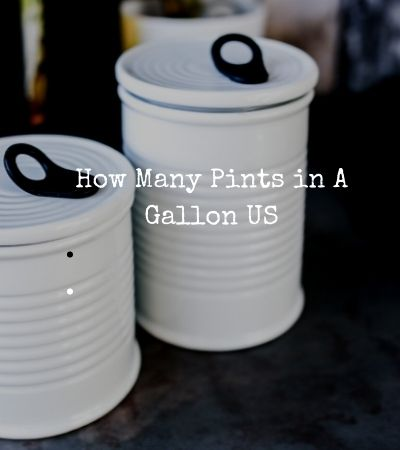# How Many Pints in a Gallon US

Contents

How Many Pints in a Gallon US: Do you want to convert pints to gallons? Or do you want to know how many pints are in a gallon?  Today we will look at simple ways to convert these units. The important thing in this conversion is understanding the conversion rates between two units of measurement. Once you understand the units, the conversion will be so easy regardless of the purpose of the conversion.

Although the US uses the imperial system, some of its unit measurements vary a little from those of the imperial system. In simpler terms, you could say that the US has its own kind of imperial system, called “US customary units.”

## How Many Pints in a Gallon

Pints in a gallon

1 US liquid gallon = 8 US liquid pints

2 US liquid gallon = 16 US liquid pints

1 Imperial Gallon (imp gal) = 9.6076 US liquid Pint (US pt)

2 Imperial Gallon (imp gal) =19.2152 US liquid pint (US pt)

In this case, if you want to know how many US pints are in a 1 US liquid gallon you have to multiply the number of gallons by 8. On the other hand, to know how many US liquid pints are in 1 imperial gallon you multiply the number of imperial gallons by 9.6076 pints.

For example

If you imported any type of liquid indicating 20 imp gal, this means the number of imperial gallons are 20. To convert these 20 imperial gallons to US pints you will multiply 20 by 9.6076 i.e (20 * 9.6076= 192.152 US pt)

## How Many Half Pints in A Gallon1 US liquid gallon = 16 US liquid half pints (US pt)

2 US liquid gallons = 32 US liquid half-pints (US pt)

1 Imperial Gallon (imp gal) = 19.2152 half US liquid Pint (US pt)

2 Imperial Gallon (imp gal) = 38.4304 half US liquid Pint (US pt)

Once you know how many (US pt) are in 1 (imp gal) i.e 1 (imp gal) is 9.6076 many (US pt), it will be easy to know how many half pints are in gallon (imp gal).

For example

If you imported a liquid indicating 40 imp gal, this means the number of imperial gallons are 40. To convert these 40 imperial gallons to half US pints you will multiply 40 by 9.6076 by 2 i.e (40 * 9.6076 *2 = 768.608 half US pt)

Find Also: How Many Tablespoons Are in an Ounce

## How Many Milliliters in a Pint

1 US pint  is 473.176 ml

1 imperial pint is 568.261 ml

## Imperial pint vs US pint

Is imperial pint equal to US pint?

The simple answer to this question is that US pint is not the same as UK pint. The US Customary pint is 473.176 milliliters while the Imperial pint is 568.261 ml. In this case, the US pint is smaller that is why while converting the imperial gallons to US pints you get many number of pints compared to when converting US gallons to US pints. i.e.

1 Imperial Gallon (imp gal) = 9.6076 US liquid Pint (US pt) vs 1 US liquid gallon = 8 US liquid pints

## How Many Pints in a Quart US

1 Imperial Quart (imp qt)= 2.4019 US pt

1 US liquid Quart (US qt) = 2 US pt

## How Many Pints in A Liter

1 US pint = 0.473176 liters

1 imperial pint =0.568261 liters

Or

1 liter = 1.75975 imperial pint

1 liter =2.11337 US Pint

## How Many Pints in A Cup

1 Imperial Cup = 0.6004 US pints

1 US legal Cup= 0.5072 US pints

Read Also: 1976 2 dollar bill value

Conclusion: How Many Pints in A Gallon?

The important thing if you want to how many pints are in an imperial gallon, cup or liter is knowing the number of measuring units in each case. Compared to imperial units, the US units are slightly smaller if you are converting to a larger unit.